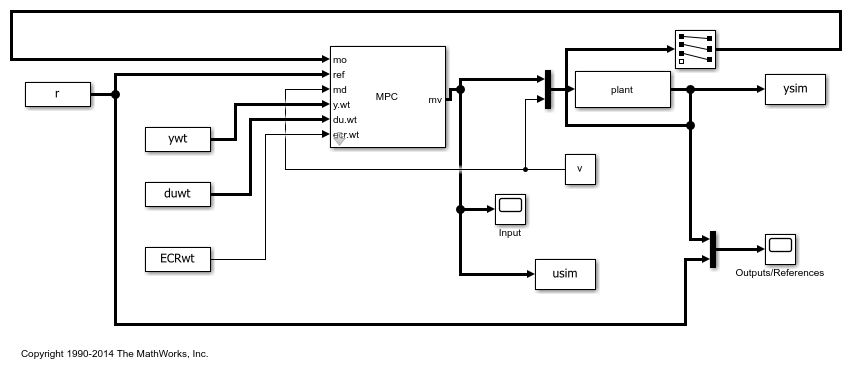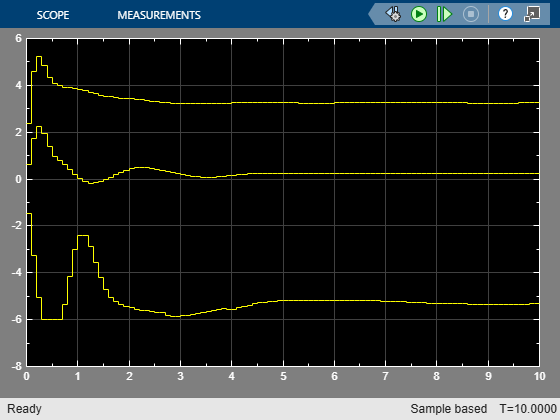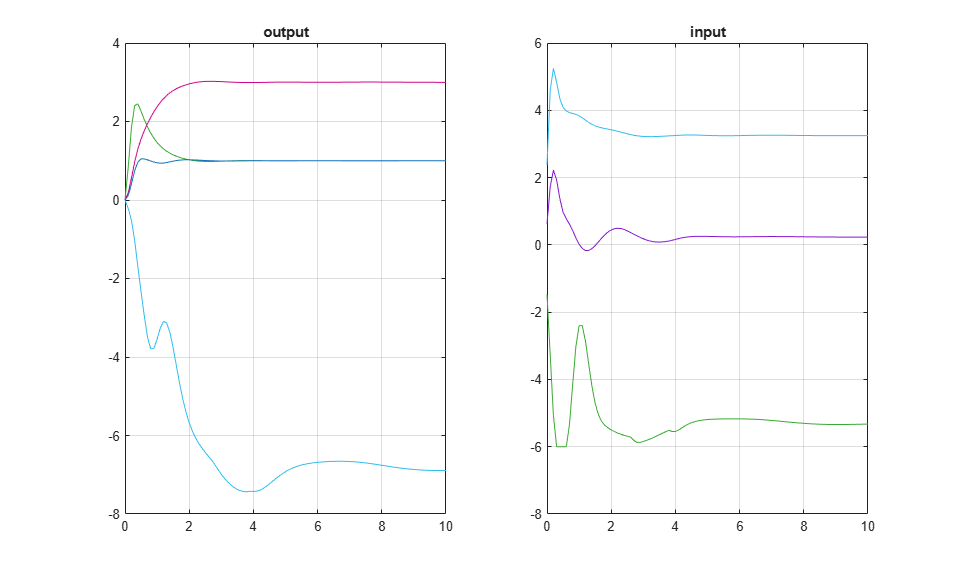# Tuning MPC Controller Weights at Run-Time

This example shows how to vary the weights on outputs, inputs, and ECR slack variable for soft constraints at run-rime, using either Simulink® or `mpcmove`.

The weights specified in the MPC object are overridden by the weights supplied to the MPC Controller block. If a weight signal is not connected to the MPC Controller block, then the corresponding weight is the one specified in the MPC object.

### Define Plant Model

Define a multivariable discrete-time linear system with no direct I/O feedthrough, and assume input #4 is a measured disturbance and output #4 is unmeasured.

```Ts = 0.1; % sampling time plant = tf({1,[1 1],5,2;3,[1 5],1,0;0,0,1,[1 1];2,[1 -1],0,0},... {[1 1 1],[1 3 4 5],[1 10],[1 5]; [1 1],[1 2],[1 2 8],[1 1]; [1 2 1],[1 3 1 1],[1 1],[1 2]; [1 1],[1 3 10 10],[1 10],[1 1]}); plant = c2d(ss(plant),Ts); plant.D = 0; % display size of the plant. size(plant) ```
```State-space model with 4 outputs, 4 inputs, and 17 states. ```

### Design MPC Controller

Specify input and output signal types.

```plant = setmpcsignals(plant,'MD',4,'UO',4); % Create the controller object with sampling period, prediction and control % horizons: p = 20; % Prediction horizon m = 3; % Control horizon mpcobj = mpc(plant,Ts,p,m); % note that default weights are assumed on inputs, input rates, and outputs ```
```-->Assuming unspecified input signals are manipulated variables. -->Assuming unspecified output signals are measured outputs. -->The "Weights.ManipulatedVariables" property of "mpc" object is empty. Assuming default 0.00000. -->The "Weights.ManipulatedVariablesRate" property of "mpc" object is empty. Assuming default 0.10000. -->The "Weights.OutputVariables" property of "mpc" object is empty. Assuming default 1.00000. for output(s) y1 y2 y3 and zero weight for output(s) y4 ```

Specify MV constraints.

```mpcobj.MV(1).Min = -6; mpcobj.MV(1).Max = 6; mpcobj.MV(2).Min = -6; mpcobj.MV(2).Max = 6; mpcobj.MV(3).Min = -6; mpcobj.MV(3).Max = 6; ```

### Define Time-Varying Signals using Structure Format

Define reference signal.

```Tstop = 10; ref = [1 0 3 1]; r = struct('time',(0:Ts:Tstop)'); N = numel(r.time); r.signals.values=ones(N,1)*ref; ```

Define measured disturbance.

```v = 0.5; ```

OV weights are linearly increasing with time, except for output #2 that is not weighted.

```ywt.time = r.time; ywt.signals.values = (1:N)'*[.1 0 .1 .1]; ```

MV rate weights are decreasing linearly with time.

```duwt.time = r.time; duwt.signals.values = (1-(1:N)/2/N)'*[.1 .1 .1]; ```

ECR weight increases exponentially with time.

```ECRwt.time = r.time; ECRwt.signals.values = 10.^(2+(1:N)'/N); ```

Start simulation.

```mdl = 'mpc_onlinetuning'; open_system(mdl); % Open Simulink(R) Model sim(mdl); % Start Simulation ```
```-->Assuming output disturbance added to measured output channel #1 is integrated white noise. -->Assuming output disturbance added to measured output channel #2 is integrated white noise. -->Assuming output disturbance added to measured output channel #3 is integrated white noise. -->The "Model.Noise" property of the "mpc" object is empty. Assuming white noise on each measured output channel. ```### Simulate Using MPCMOVE Command

Define real plant and MPC state object.

```[A,B,C,D] = ssdata(plant); x = zeros(size(plant.B,1),1); % Initial state of the plant xmpc = mpcstate(mpcobj); % Handle to state of the MPC controller ```

Store the closed-loop MPC trajectories in arrays YY,UU,XX.

```YY = []; UU = []; XX = []; ```

Use `mpcmoveopt` object to provide weights at run-time.

```options = mpcmoveopt; ```

Start simulation.

```for t = 0:N-1, % Store states XX = [XX,x]; %#ok<*AGROW> % Compute and store plant output (no feedthrough from MV to Y) y = C*x+D(:,4)*v; YY = [YY;y']; % Obtain reference signal ref = r.signals.values(t+1,:)'; % Update |mpcmoveopt| object with run-time weights options.MVRateWeight = duwt.signals.values(t+1,:); options.OutputWeight = ywt.signals.values(t+1,:); options.ECRWeight = ECRwt.signals.values(t+1,:); % Compute and sore control action u = mpcmove(mpcobj,xmpc,y(1:3),ref,v,options); UU = [UU;u']; % Update plant states x = A*x + B(:,1:3)*u + B(:,4)*v; end ```

Plot and Compare Simulation Results.

```figure(1); clf; subplot(121) plot(0:Ts:Tstop,[YY ysim]) grid title('output') subplot(122) plot(0:Ts:Tstop,[UU usim]) grid title('input') ```Compare input and output signals.

```norm(UU-usim) norm(YY-ysim) % results are identical except for numerical errors. ```
```ans = 4.2786e-11 ans = 8.8298e-12 ```
```bdclose(mdl); ```